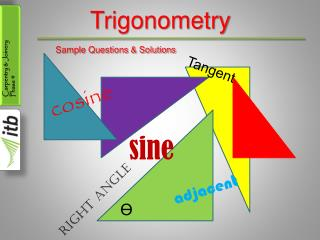Download PresentationTrigonometry

# Trigonometry - PowerPoint PPT PresentationDownload Presentation## Trigonometry

- - - - - - - - - - - - - - - - - - - - - - - - - - - E N D - - - - - - - - - - - - - - - - - - - - - - - - - - -
##### Presentation Transcript

1. Trigonometry Sample Questions & Solutions Tangent cosine sine adjacent Right Angle Ө

2. Pythagoras Theorem • Given a = 3.25m & b = 3.45m calculate the length of c • a² + b² = c² • 3.25² + 3.45² = c² • 10.5635 + 11.9025 = c² • 22.466 = c² • c = √22.466 • c = 4.74m Answer c a b

3. Question (c) (b) (a) A A (e) A A (f) (d) A A Copy each of the triangles below and label each of the sides and the angle.

4. Answer (c) (b) (a) A A (e) A A (f) (d) A A Copy each of the triangles below and label each of the sides and the angle. adj hyp opp opp hyp opp hyp adj adj adj adj opp hyp opp adj opp hyp hyp

5. Question • Use your calculator to answer to 4 decimal places (a) cos 25° (b) sin 50° (c) tan 34°

6. Answer • Use your calculator to answer to 4 decimal places (a) cos 25° (b) sin 50° (c) tan 34° • (a) cos 25 = 0.906307787 = 0.9063 • (b) sin 50 = 0.766044443 = 0.7660 • (c) tan 34 = 0.674508516 = 0.6745

7. Question • Use your calculator to find the angles to the nearest degree (a) sin A = 0.83 (b) cos B = ¾ (c) tan A = 5/7

8. Answer • Use your calculator to find the angles to the nearest degree (a) sin A = 0.83 (b) cos B = ¾ (c) tan A = 5/7 • (a) 2ndF, sin, .83 = 56.098738 = 56° • (b) 2ndF, cos, a/b, 3 ↓ 4 = 41.40962211 = 41° • (c) 2ndF, tan, a/b, 5 ↓ 7 = 35.53767779 = 36°

9. Solving Right-angle Triangles • In the triangle below, find the length of b • The side we must find is the adjacent (b) • We have the hypotenuse c = 10m • The ratio that uses adj. & hyp. is cos • So cos 60° = = • 0.5 = • b = 10(0.5) • b = 5m Answer adjacent hypotenuse b 10 b 10 c = 10m a 60° b

10. Solving Right-angle Triangles • Find the angle A to the nearest degree • We have the opposite & the adjacent so we use tan ratio • Tan A = = • Tan A = • Use calculator 2ndF, tan, a/b, 4 ↓ 3 = • A = 53.1301 • A = 53° Answer Opposite adjacent 4 3 4 3 A° 3m adjacent 4m opposite

11. Solving Right-angle Triangles • Find the side c (hyp.) to 2 decimal places • We are given angle & opposite to find hyp. • sin 40° = • 0.6428 = • 0.6428 x c = 15 • c = • c = 23.34 Answer 15 c 15 c 15 0.6428 c 15m 40° b

12. Solving Right-angle Triangles • Given a section through a roof with 2 unequal pitches, calculate (a) the rise of the roof (b) the length of rafter c (c) the span b 5.42m c 40° 30° b

13. Solving Right-angle Triangles • This question may seem complicated but we can simplify it by using our knowledge of right-angle triangles • Firstly, divide triangle into 2 right-angled triangles (using the rise line) 5.42m c rise 40° 30° b

14. Solving Right-angle Triangles • On the larger triangle we have the angle 30° & the hypotenuse 5.42m so we can use ratio sin 30° to find the opposite (rise) Sin = (soh) opposite hypotenuse 5.42m c 40° 30° b

15. Solving Right-angle Triangles opposite hypotenuse • Sin 30°= • 0.5 = • 0.5 x 5.42 = rise • rise = 2.71m Answer (a) rise 5.42 5.42m c 40° 30° b

16. Solving Right-angle Triangles • So on the smaller triangle we now have the opposite (2.71m) and the angle 40° • So to find adjacent (part of span) we use Tan Tan 40° = 0.839 = 0.839 x adj. = 2.71 adj. = 2.71 ÷ 0.839 = 3.23m Opposite adjacent 2.71 adjacent 5.42m c 40° 30° b adj.

17. Solving Right-angle Triangles • Using Pythagoras we can find c • a² + b² = c² • 2.71² + 3.23² = c² • 7.3441 + 10.4329 = c² • c² = 17.777 • c = √17.777 • c = 4.216m Answer (b) 5.42m c 2.71m 40° 30° 3.23m b

18. Solving Right-angle Triangles • Using Pythagoras we can find the rest of the span i.e. the base of the larger triangle • a² + b² = c² • 2.71² + b² = 5.42² • 7.344 + b² = 29.376 • b² = 29.376 – 7.344 • b² = 22.032 • b = √22.032 • b = 4.694m 5.42m c 2.71m 40° 30° b 3.23m

19. Solving Right-angle Triangles So 4.694 + 3.23 = span Span = 7.924m Answer (c) 5.42m c 2.71m 40° 30° b 3.23m